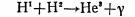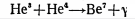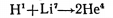Formulas of proton–proton chain reactions (pp-cycle)

Proton–proton chain is a cycle of thermonuclear reactions occurring in stars as a result of which hydrogen is converted into helium. According to the perceptions of modern nuclear physics, pp -cycle is the main source of the sun energy.

 Reaction: Verification: Correct: H21 + p11 → He32 + γ 45 + 12 → 57 + 3 57≠60 H21 + p11 → He32 57=57Reaction: Verification: Correct: He32 + He42 → Be74 + γ 57 + 90 → 147 + 3 147≠150 He32 + He42 → Be74 147=147Reaction: Verification: Li73 + p11 → He42 + He42 168 + 12 → 90 + 90 180=180References: Путилов К.А., Фабрикант В.А. Курс физики. В 3-х томах. Том 3. [Putilov, K.A, Fabrikant V.A. Course of Physics. In 3 volumes. Vol. 3] Moscow: FIZMATGIZ, 1963. P. 626 [In Russian]